# plotnineplotnine is an implementation of a grammar of graphics in Python, it is based on ggplot2. The grammar allows users to compose plots by explicitly mapping data to the visual objects that make up the plot.

Plotting with a grammar is powerful, it makes custom (and otherwise complex) plots easy to think about and then create, while the simple plots remain simple.

To find out about all building blocks that you can use to create a plot, check out the documentation. Since plotnine has an API similar to ggplot2, where we lack in coverage the ggplot2 documentation may be of some help.

## Example

```from plotnine import *
from plotnine.data import mtcars```

Building a complex plot piece by piece.

1. Scatter plot

```(ggplot(mtcars, aes('wt', 'mpg'))
+ geom_point())```
2. Scatter plot colored according some variable

```(ggplot(mtcars, aes('wt', 'mpg', color='factor(gear)'))
+ geom_point())```
3. Scatter plot colored according some variable and smoothed with a linear model with confidence intervals.

```(ggplot(mtcars, aes('wt', 'mpg', color='factor(gear)'))
+ geom_point()
+ stat_smooth(method='lm'))```
4. Scatter plot colored according some variable, smoothed with a linear model with confidence intervals and plotted on separate panels.

```(ggplot(mtcars, aes('wt', 'mpg', color='factor(gear)'))
+ geom_point()
+ stat_smooth(method='lm')
+ facet_wrap('~gear'))```
5. Make it playful

```(ggplot(mtcars, aes('wt', 'mpg', color='factor(gear)'))
+ geom_point()
+ stat_smooth(method='lm')
+ facet_wrap('~gear')
+ theme_xkcd())```

## Installation

Official release

```# Using pip
\$ pip install plotnine         # 1. should be sufficient for most
\$ pip install 'plotnine[all]'  # 2. includes extra/optional packages

# Or using conda
\$ conda install -c conda-forge plotnine```

Development version

`\$ pip install git+https://github.com/has2k1/plotnine.git`

## Contributing

Our documentation could use some examples, but we are looking for something a little bit special. We have two criteria:

1. Simple looking plots that otherwise require a trick or two.
2. Plots that are part of a data analytic narrative. That is, they provide some form of clarity showing off the geom, stat, ... at their differential best.

If you come up with something that meets those criteria, we would love to see it. See plotnine-examples.

If you discover a bug checkout the issues if it has not been reported, yet please file an issue.

And if you can fix a bug, your contribution is welcome.

We are re-licensing plotnine to MIT. It was only licensed as GPL since it was derived from ggplot2 which was GPL at the time, and, now that ggplot2 is MIT, we can relicense plotnine.

To make this change to plotnine, we need the approval of all copyright holders, which I have found by reviewing contributions from all contributors made after the January 2017 change to GPLv2.

@akdor1154, @Alyetama, @astrocorgi, @CarlosGrohmann, @ChickenProp, @dulacp, @Fischmiep, @gokceneraslan, @hugovk, @hyiltiz, @JarnoRFB, @jdanbrown, @jeroenjanssens, @joshhartmann11, @jsoma, @jsspencer, @khaeru, @kingishb, @kngwyu, @machow, @stillmatic, @stonebig, @tabedzki, @thomasjpfan, @tr8dr, @TyberiusPrime, @yejianye, would you permit us to re-license plotnine with the MIT license? If so, please comment "I agree" below.

enhancement
opened by has2k1 28

This pull requests adds 'annotation_logticks()' similar to the ggplot2 one: https://ggplot2.tidyverse.org/reference/annotation_logticks.html

This adds log-10 'log ticks' as an annotation similar to a rug to the plot.

Looks like this: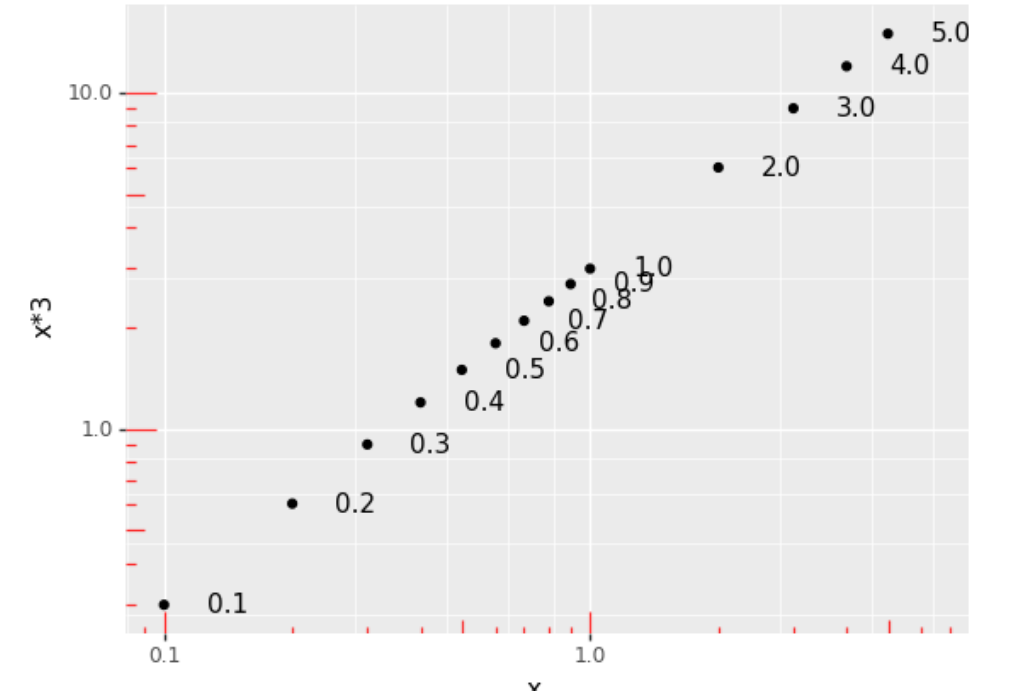Example usage:

``````import pandas as pd
import plotnine
df = pd.DataFrame({"x": [0.1, 0.2, 0.3, 0.4, 0.5, 0.6, 0.7, 0.8, 0.9, 1.0,
2, 3, 4, 5]})
p = plotnine.ggplot(df, plotnine.aes('x','x*3'))
p += plotnine.geom_point()
p += plotnine.geom_text(plotnine.aes(label='x'),
ha='left', nudge_x=.1)
p += plotnine.scale_y_log10()
p += plotnine.scale_x_log10()
#p += plotnine.geom_rug(sides='lb', width=2, alpha=0.5) #  to check correctness
p += plotnine.geoms.annotation_logticks(sides='lb', color='red',
alpha=0.8, width=(1.2,.75, 0.4),
linetype='solid')

p
``````

Right now this is not perfect. For one, it's a 'fake geom' that ignores it's data.

And I've failed to detect when the axis are not log10, and it then will plot misleading tick marks. Can't for the life of me figure out how the geom could detect it though - coord.is_linear is always true.

opened by TyberiusPrime 23
• #### input validation on geom_violin(draw_quantiles)

This PR checks, normalizes and documents the draw_quantiles parameter on geom_violin.

It now accepts any iterable of floats, or a single float (to be feature compatible with ggplot2). They get converted into a list internally.

If it's not valid (ie. not float, [float] or values outside of 0..1 (exclusive), a ValueError is raised when calling geom_violin.

Previously, the following happened:

• draw_quantiles = True -> Exception in drawing `TypeError: object of type 'bool' has no len()`
• draw_quantiles = 0.5 -> Exception in drawing: `TypeError: object of type 'float' has no len()`
• draw_quantiles = np.array([0.5]) -> ok
• draw_quantiles = np.array([0.3, 0.5]) -> exception in drawing: `ValueError: The truth value of an array with more than one element is ambiguous. Use a.any() or a.all()`
• draw_quantiles = 0 -> interpreted as False
• draw_quantiles = [0.0] -> exception in drawing: `ValueError: A value in x_new is below the interpolation range.`
• draw_quantiles = [1.0] -> exception in drawing: `ValueError: A value in x_new is above the interpolation range.`
• draw_quantiles = [1.25] -> exception in drawing: `ValueError: A value in x_new is above the interpolation range.`

These all now either work, or throw a sensible exception `ValueError("draw_quantiles must be a float or an iterable of floats (>0.0; < 1.0)")` much closer to the actual erroneous call.

opened by TyberiusPrime 17
• #### Use aliased imports in the gallery

I would like to suggest using "import plotnine as p9" (or something like that) in the gallery code instead of "from plotnine import *", and then using "p9" as a prefix for all identifiers defined by plotnine. That would make it much clearer which of them come from plotnine and which don't.

enhancement
opened by jaccovanschaik 16
• #### introduced annotation_alternating_backgrounds

This PR introduces an annotation that adds 'alternating' backgrounds, which is useful to increase readability in tight jitter plots on categorical x-axis data. Example:

``````import plotnine as p9
from plotnine.data import mtcars
g = p9.ggplot(mtcars.assign(
gear = pd.Categorical(mtcars.gear),
am = pd.Categorical(mtcars.am)
))
g += p9.geom_jitter(p9.aes("gear", 'wt', color='am'), width=.45, height=0)
g += p9.annotation_alternating_background(fills=['grey','darkgrey'],
alpha=.5)
g += p9.scale_color_manual(['red','blue'])
``````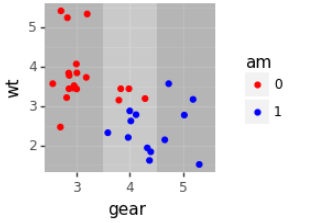Supports coord_flip: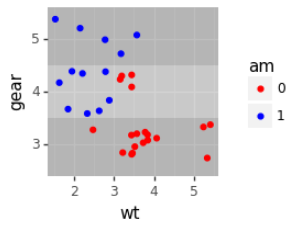And more than 2 colors, and does something sensible with non-discrete axis (ie. change shade halfway between labels)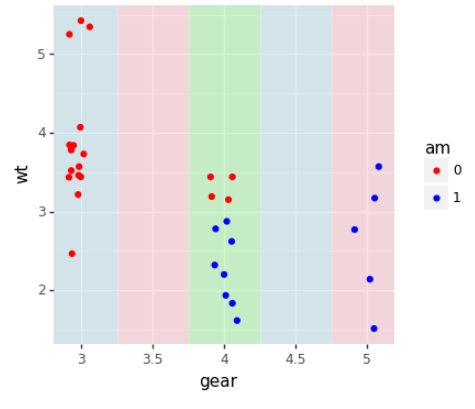opened by TyberiusPrime 15

Here's a first implementation of adust_text using a position - see #218 I have extended the position to allow a post-drawing callback, and positions can now define a list of geoms that they work with.

While this enables the basic use case, there are some kinks we should probably talk with upstream about:

``````(p9.ggplot(mtcars, p9.aes('mpg','disp')) + p9.geom_point()
+ p9.geom_text(p9.aes(label='name'), color='blue', position = p9.position_adjust_text(), size=7)
)
``````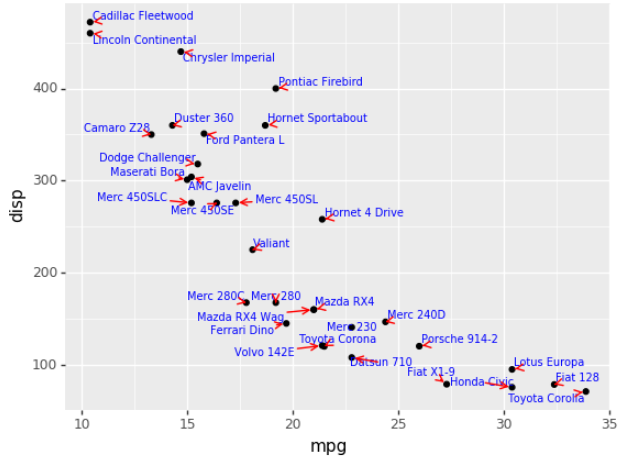1. Two sets of points and labels don't work well:
``````g = (p9.ggplot(mtcars, p9.aes('mpg','disp'))
+ p9.geom_point()
+ p9.geom_text(p9.aes(label='name'), color='blue',
'arrowprops': {'arrowstyle': '-', 'color': 'blue'}
}), size=7)
+ p9.geom_point(p9.aes('mpg+1','disp'), color='green')
+ p9.geom_text(p9.aes(x='mpg+1', label='name'), color='green',
'arrowprops': {'arrowstyle': '-', 'color': 'green'}
}), size=7)
)
g
``````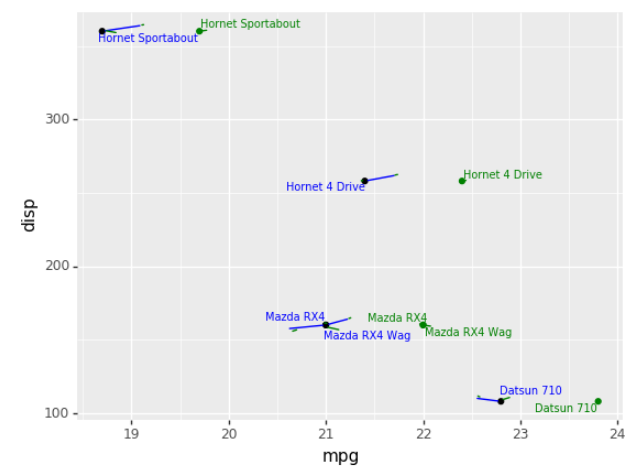(I think it's an issue with both set's of text labels being drawn on the same ax, and accordingly the second call sees the first set again. Will have to debug.)

1. labels and arrows interact badly
``````(p9.ggplot(mtcars, p9.aes('mpg','disp')) + p9.geom_point()
+ p9.geom_label(p9.aes(label='name'), color='blue', position = p9.position_adjust_text(), size=7)
)
``````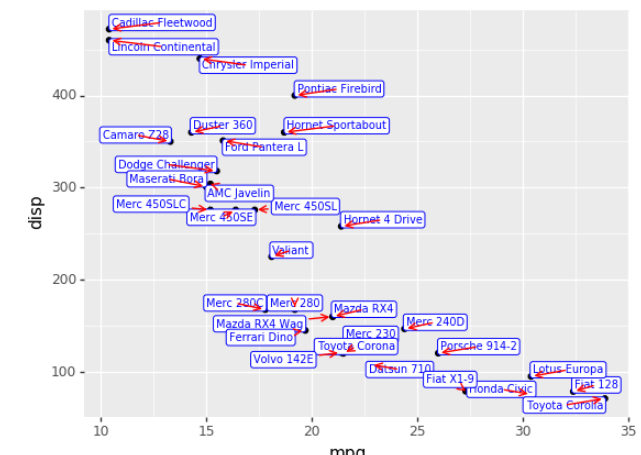1. no geom_point() -> broken plot
``````(p9.ggplot(mtcars, p9.aes('mpg','disp'))
# + p9.geom_point()
+ p9.geom_text(p9.aes(label='name'), color='blue', position = p9.position_adjust_text(), size=7)
)
``````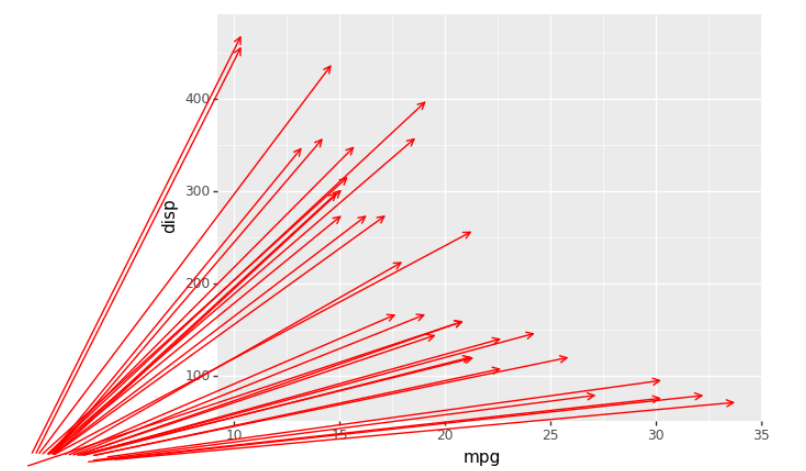3b) geom_point() after geom_text(position_adjust_text) > broken plot

``````(p9.ggplot(mtcars, p9.aes('mpg','disp'))
+ p9.geom_text(p9.aes(label='name'), color='blue', position = p9.position_adjust_text(), size=7)
+ p9.geom_point()
)
``````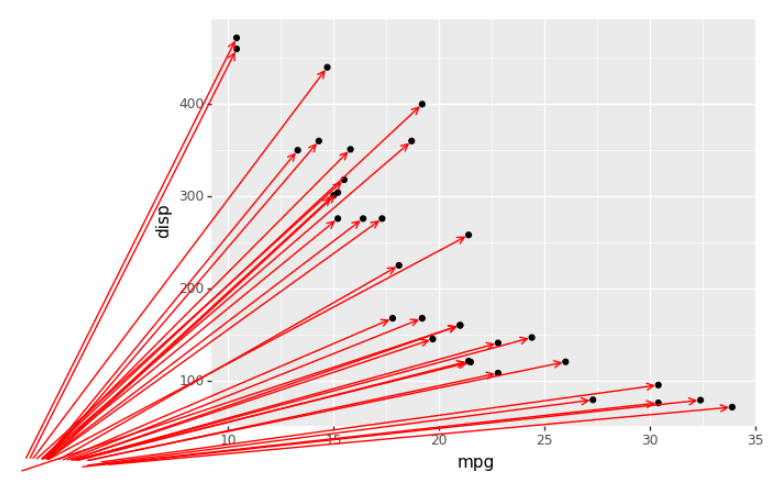Also, I note that the adjustment is not fast - it takes a few seconds ever for these 'simple' plots.

opened by TyberiusPrime 15
• #### New matplotlib warnings - when using plotnine 0.4.0

I am getting some new warnings when using plotnine. My point is - let's make sure, plotnine adapts to the latest matplotlib changes.

USED VERSIONS & PLATFORMS: OS: Win10 64-bit Anaconda Python: 3.6.6

matplotlib.version '3.0.0' plotnine.version '0.4.0'

WARNINGS EXAMPLES: C:\Users\stesim\Anaconda3\envs\py36\lib\site-packages\plotnine\coords\coord_cartesian.py:31: MatplotlibDeprecationWarning: The Bunch class was deprecated in Matplotlib 3.0 and will be removed in 3.2. Use types.SimpleNamespace instead. self.limits = Bunch(xlim=xlim, ylim=ylim)

C:\Users\stesim\Anaconda3\envs\py36\lib\site-packages\plotnine\facets\layout.py:147: MatplotlibDeprecationWarning: The Bunch class was deprecated in Matplotlib 3.0 and will be removed in 3.2. Use types.SimpleNamespace instead. return Bunch(x=xsc, y=ysc)

C:\Users\stesim\Anaconda3\envs\py36\lib\site-packages\plotnine\coords\coord.py:144: MatplotlibDeprecationWarning: The Bunch class was deprecated in Matplotlib 3.0 and will be removed in 3.2. Use types.SimpleNamespace instead. y=panel_params['y_range'])

enhancement invalid
opened by stefansimik 14
• #### Allow user to change relative width or height of facets

This change allows a user of facet_grid() to specify an optional:

• space={'y': `<ratios>`}: setting the relative height of vertical facets
• for example facet_grid("pane ~ .", scales='free_y', space={'y': [2,1,1]}. This would make the top facet height 2x that of the 2nd and 3rd facet
• space={'x': `<ratios>`}: setting the relative width of horizontal facets
• for example space={'x': [1,2,1], ...}
• or a combination of the two:
• space={'x': [1,2,1], 'y': [2,1,1]}.

A similar extension exists for R ggplot(), though not in the main ggplot package. Here is an example:

``````import numpy as np
import pandas as pd
import plotnine
from plotnine import *

y = pd.Series(np.random.normal(0.0, 1.0, 400)).cumsum()
df = y.diff().fillna(0.0)
x = np.arange(0,400)

gdf = pd.concat([
pd.DataFrame({'x': x, 'y': y, 'what': 'y', 'pane': 'cumr'}),
pd.DataFrame({'x': x, 'y': df, 'what': 'df', 'pane': 'df'}),
])

(ggplot() +
geom_line(aes(x='x', y='y', color='what'), data=gdf) +
facet_grid("pane ~ .", scales="free_y", space={'y': [3,1]}))
``````

See the attached output included.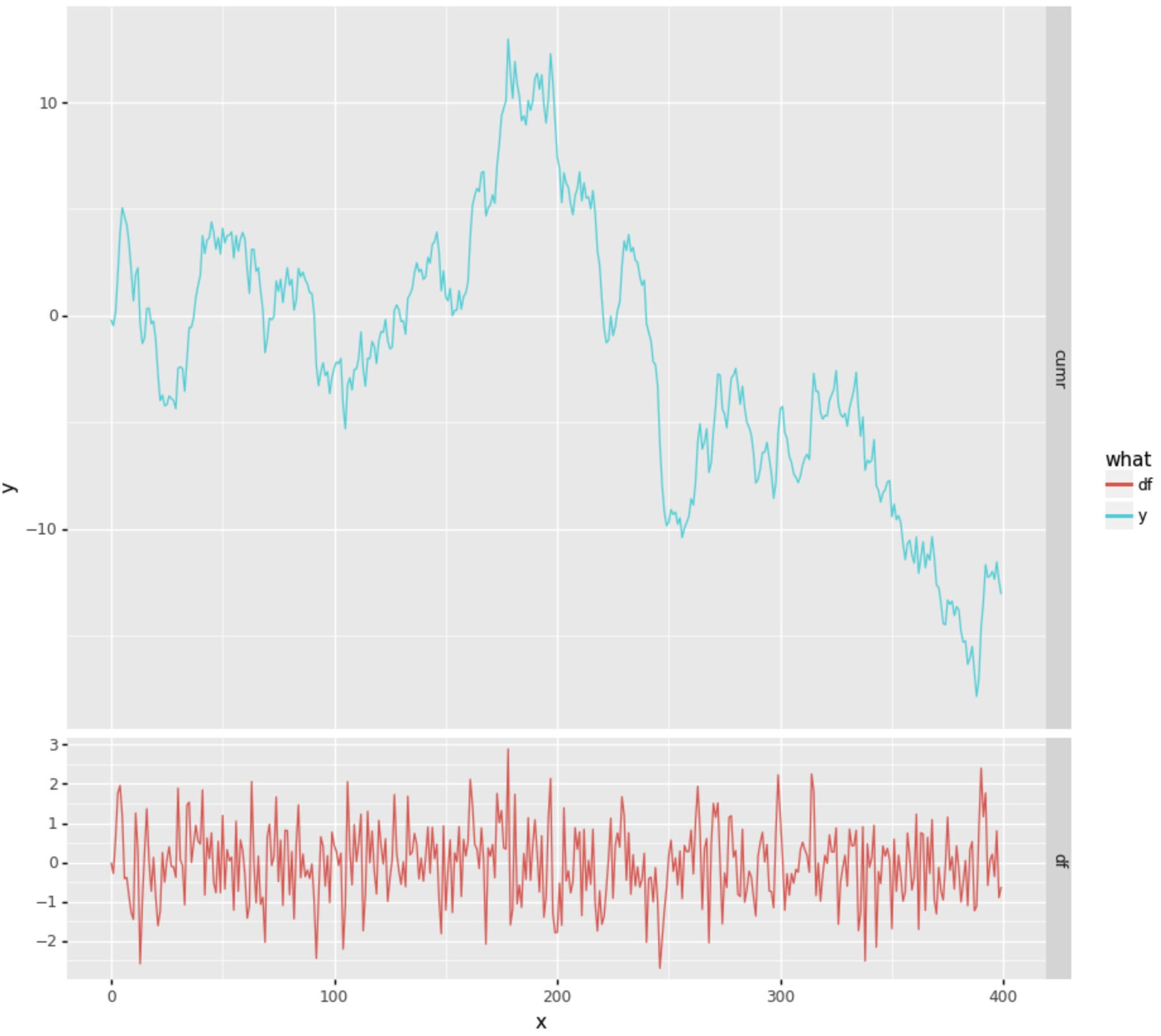opened by tr8dr 13
• #### Plotnine warnings cherry

This PR introduces a new Warning class PlotNineWarning, which is now used throughout the plotnine code base when emitting warnings

It allows the downstream to capture PlotNine warnings in a structured way.

Note that PlotNine will still emit UserWarnings from upstream - they are not being rewritten.

I've also promoted PlotNineException and PlotNineWarning to top-level objects on the module, so they're visible to the user.

opened by TyberiusPrime 13
• #### plotnine and pyqt5

Hello,

`plotnine` is a wonderful package. I am trying to figure out how to incorporate plots into a `pyqt5` application. For `matplotlib`, there are a number of examples, but with `plotnine`, I don't know where to start. Any guidance is greatly appreciated.

Thank you.

enhancement question documentation
opened by breisfeld 10
• #### Possible to hide legend?

R ggplot supports `theme(legend.position = "none")`, but plotnine doesn't appear to recognize `'none'` in `theme(legend_position='none')`.

• http://ggplot2.tidyverse.org/reference/theme.html

Is there another way to do this, or is this a feature that would need to be added?

enhancement
opened by jdanbrown 9
• #### geom_violin with facet_grid breaks scales="free"

I want to plot `geom_violins` for my data with `facet_grid` to distinguish variables and I need the scales (at least `y`) to be free.

I obtain the following result, notice the same scales for the facets: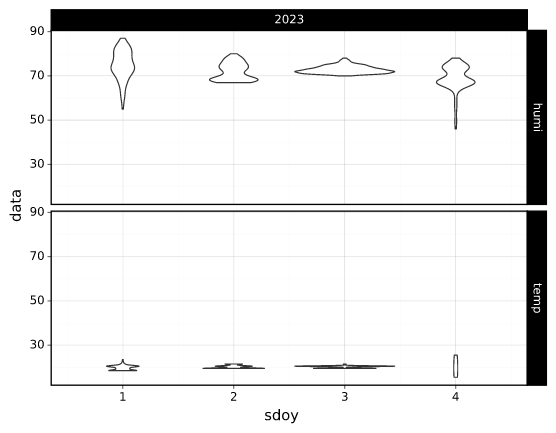with the following code where I specify `scales='free'`:

``````p = (
ggplot(df)
+ facet_grid("type ~ year", scales="free")
+ geom_violin(aes(x="sdoy", y="data", group="sdoy"))
)
``````

Also if I replace `geom_violin` by `geom_boxplot` it works: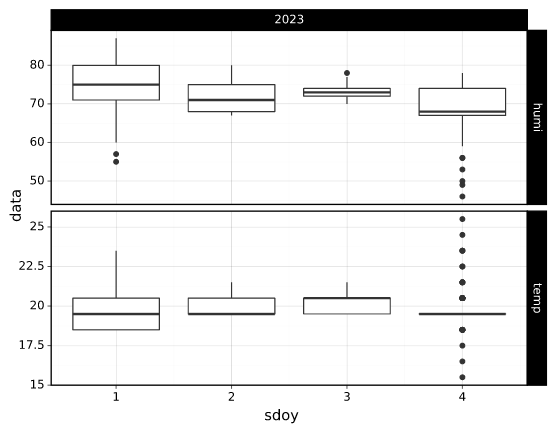I can't find were this specificity is mentioned, or is it a bug?

opened by gigaturbo 0
• #### geom_ribbon and geom_smooth problems

I've previously used geom_ribbon() and geom_smooth() without problems, but now I cannot make my old scripts work. I keep bumping to this:

2357 2358 """ -> 2360 return masked_where(~(np.isfinite(getdata(a))), a, copy=copy)

TypeError: ufunc 'isfinite' not supported for the input types, and the inputs could not be safely coerced to any supported types according to the casting rule ''safe''

All data types are correct and no inf or na values are present.

upstream-bug critical-bug
opened by laurivil 3

### Discussed in https://github.com/has2k1/plotnine/discussions/652

Originally posted by AlFontal December 12, 2022 I've been trying to add borders to colorbars to be consistent with other colorbars generated in `matplotlib`, which by default contain black borders.

I could always remove the borders on the `matplotlib` side, but I've come to quite like the aesthetics of a bordered colorbar, and I was wondering whether it's even possible to replicate that.

As an extra, `ggplot2`'s current release allows to do this by using the `frame.colour` attribute in `guide_colorbar`.

Does anyone know whether there is any way of doing this through the current `plotnine` API?

Minimal example of what I mean:

``````df = pd.DataFrame({
'value': np.random.randn(100),
'variable': np.tile(['A', 'B', 'C', 'D', 'E'], 20),
'time': np.repeat(np.arange(1, 21), 5)
})
``````
``````# in matplotlib
plt.imshow(df.pivot('time', 'variable', 'value'))
plt.colorbar()
``````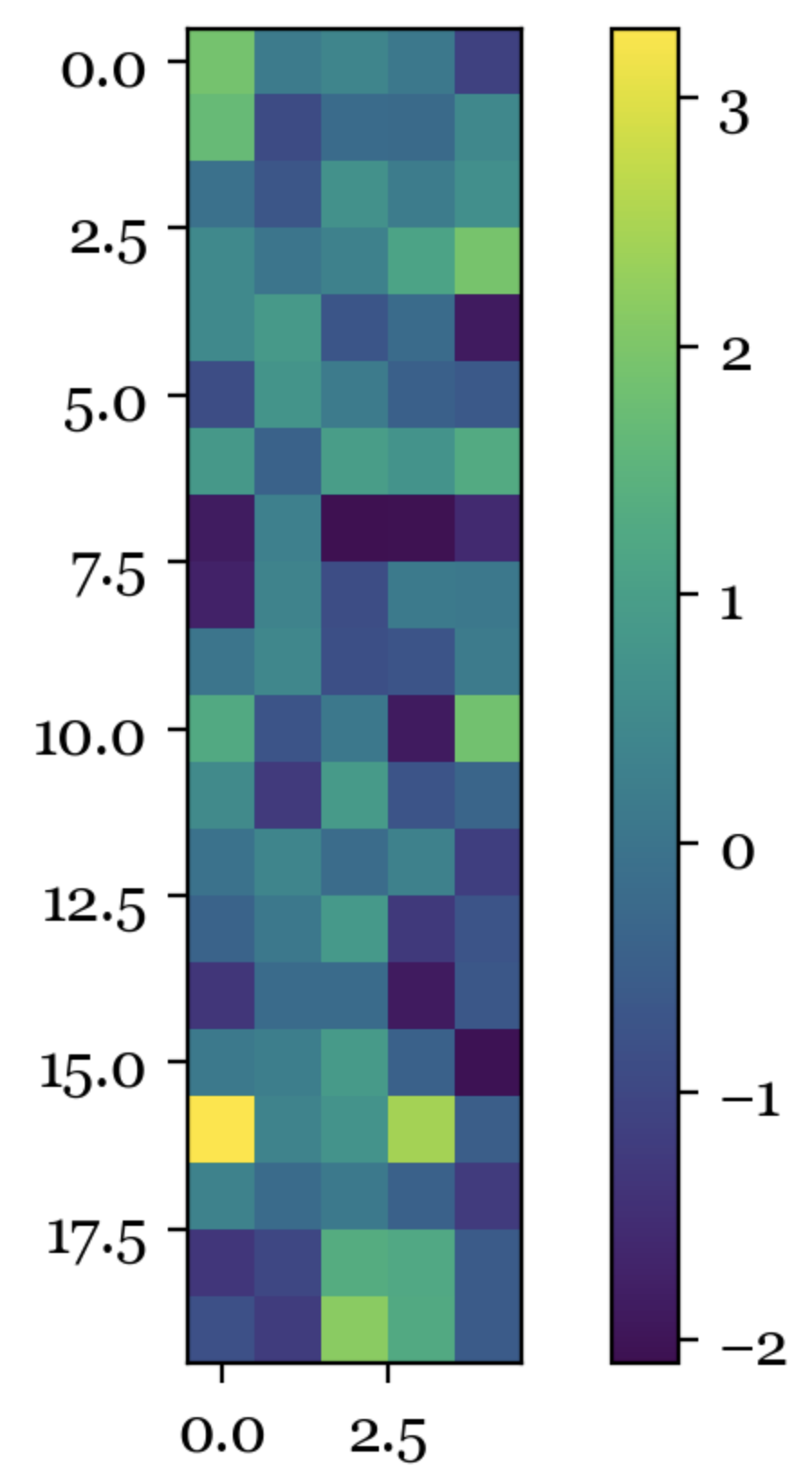``````# ggplot2 in R
ggplot(df) +
aes(variable, time, fill = value) +
geom_tile() +
scale_fill_continuous(type = 'viridis',
guide = guide_colorbar(frame.colour = 'black'))
``````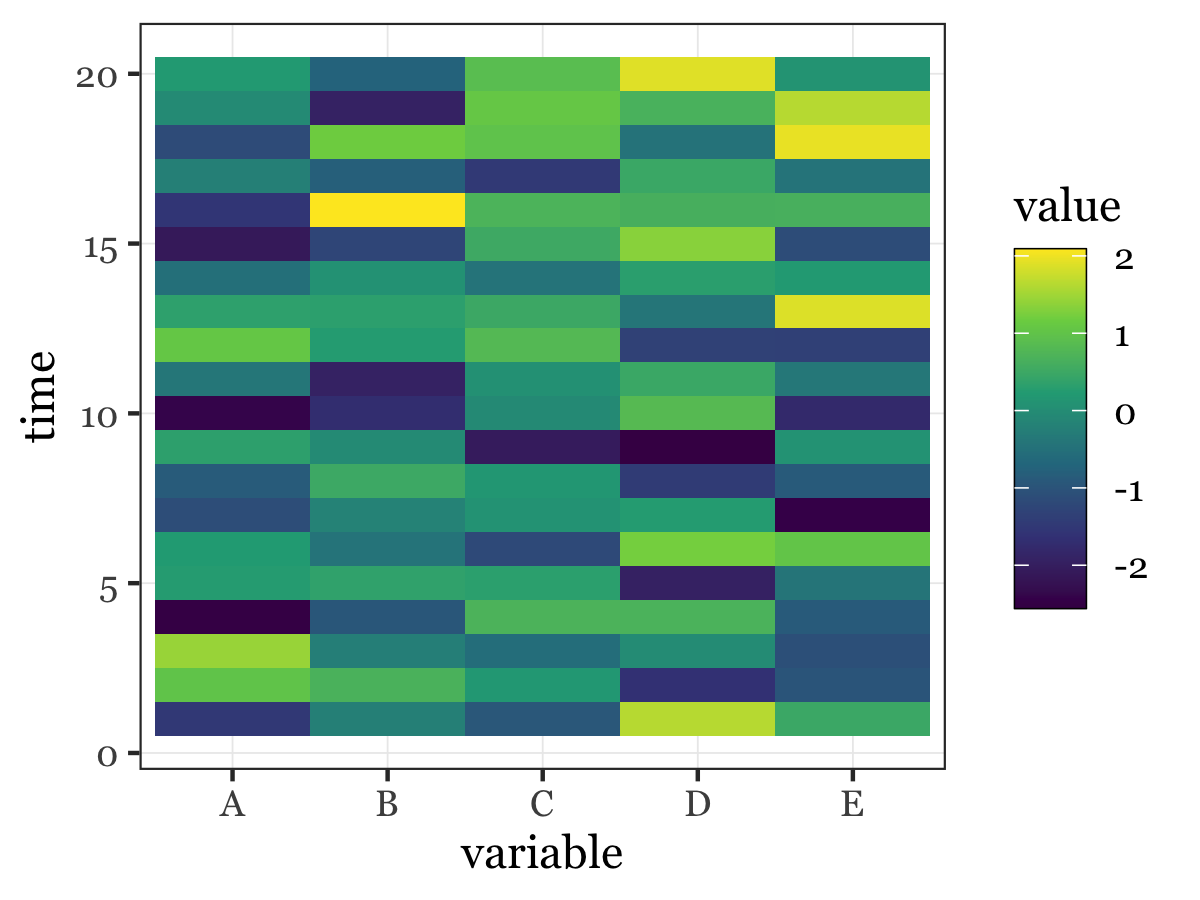feature
opened by AlFontal 0
• #### geom_text: do not eliminate NaN when format_string or parse is given

As string.format happily replaces an NaN with "nan", we forget the information that a value was missing, whereby it is no longer removed by na_rm, but the string "nan" is displayed.

opened by stettberger 0
• #### legend: use figure artist instead of axis artist

When positioning a legend in an faceted plot in an facet != ax, the legend is not drawn in front of the facets, but behind them. This behavior is different from ggplot.

Without the Patch: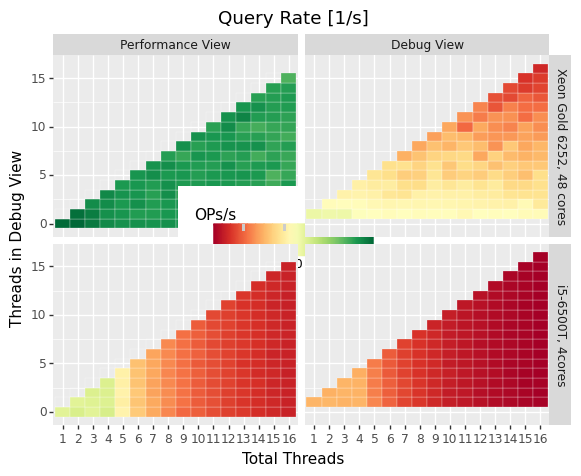With the Patch: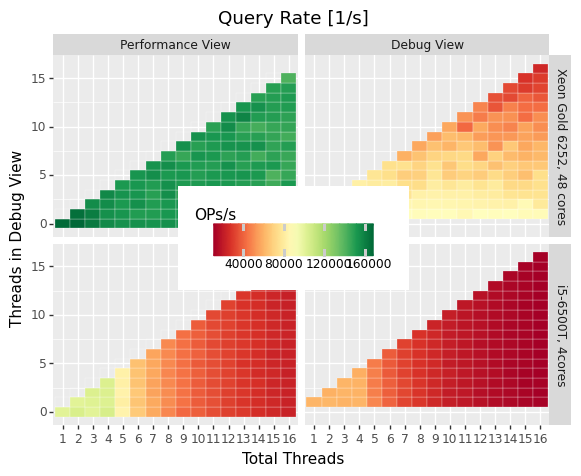opened by stettberger 2
• #### Tests fail with ImportError

Running tests in the Fedora 36 build environment with Python 3.10.7, pytest-6.2.5, py-1.11.0, pluggy-1.0.0, test_geom_bar_col_histogram.py and test_geom_bin_2d.py fail with:

``````ImportError while importing test module '/builddir/build/BUILD/plotnine-0.10.1/tests/test_geom_bar_col_histogram.py'.
Hint: make sure your test modules/packages have valid Python names.
Traceback:
tests/test_geom_bar_col_histogram.py:8: in <module>
from .conftest import layer_data
E   ImportError: attempted relative import with no known parent package
``````
``````ImportError while importing test module '/builddir/build/BUILD/plotnine-0.10.1/tests/test_geom_bin_2d.py'.
Hint: make sure your test modules/packages have valid Python names.
Traceback:
tests/test_geom_bin_2d.py:6: in <module>
from .conftest import layer_data
E   ImportError: attempted relative import with no known parent package
``````

I tried working around it by providing an empty init.py in tests/, but that was intercepted by conftest.py itself:

``````../../BUILDROOT/python-plotnine-0.10.1-2.fc36.x86_64/usr/lib/python3.10/site-packages/tests/conftest.py:30: in <module>
raise OSError(
E   OSError: The baseline image directory does not exist. This is most likely because the test data is not installed. You may need to install plotnine from source to get the test data.
``````

Running the tests in Fedora 37, which has Python 3.11.0rc2, pytest-7.1.3, pluggy-1.0.0, as is (without empty init.py) works fine.

wontfix Installation
opened by penguinpee 3

## API Changes

• How you map to calculated aesthetics has changed. Use the `~plotnine.aes.after_stat` function. The old methods `'stat(name)'` and `'..name..'` have been deprecated.

## New Features

• You can now map to aesthetics at three different stages. See `~plotnine.aes.aes`, `~plotnine.aes.after_stat`, `~plotnine.aes.after_scale` and `~plotnine.aes.stage`.
• `~plotnine.geoms.geom_violin` gained the a new parameter `style` with which you can draw half violin (density curve on one side and flat on the other).
• Added `~plotnine.geoms.geom_raster`.
• `geoms` gained new parameter `raster` for the `~plotnine.layer.Layer`. You can use it to rasterize any layer when the resulting plot is of vector format e.g. `pdf`.

## Bug Fixes

• Fixed issue where some plots with a colorbar would fail for specific themes. (`424`)
• Fixed `~plotnine.geoms.geom_map` to plot `MultiLineString` geom types.
• Fixed `~plotnine.geoms.geom_text` to allow any order of `mapping` and `data` positional arguments.
• Fixed bug were the plotted image may have ignored theming that relied on some Matplotlib rcParams. (`451`)
• Fixed the `weight` aesthetic in `~plotnine.geoms.geom_boxplot`, previously ignored it is now recognised. (`438`)
• Fixed `~plotnine.geoms.annotation_logticks` and `~plotnine.geoms.annotation_stripes` to work without global data and aesthetics. (`469`)
• Fix `~plotnine.scales.scale_shape_discrete` when print many unfilled shapes not to assign the same shapes to more than one group. (`473`)
• Fixed bug in `~plotnine.stats.stat_ellipse` where the center of the ellipse assuming a multivariate t-distribution was incorrectly calculated. (`493`)
• Fixed calculation of `ndensity` in `~plotnine.stats.stat_bin`. (`494`)

## Enhancements

• Manual scales now match the values of the breaks if the breaks are given. (`445`)

• Using `print` to show a ggplot object will not show the hash (`<ggplot: ...>`) anymore. There is now a difference between `repr(p)` and `str(p)`. (`453`)

• Added option to for the `base_family` of a theme, now you can set it once with and have it be applied to all themes. (`436`)

``````from plotnine.options import set_option
set_option('base_family', 'Comic Sans MS')
``````
• You can now add `None` to a ggplot, doing so returns a copy of the the ggplot object. (`474`)

• Better handling of multiline facet labels. (`484`)

Source code(tar.gz)
Source code(zip)
• #### v0.7.1(Aug 5, 2020)

v0.7.1 is a small bugfix and maintenance release. See the official changelog for details. A copy of the changes is also copied below.

## Bug Fixes

• Fixed issue where a plot has no data and the geoms have no data, but the mappings are valid. (`404`)
• Fixed `preserve='single'` in `plotnine.positions.position_dodge` and `plotnine.positions.position_dodge2` to work for geoms that only have `x` aesthetic and not `xmin` and `xmax` e.g `plotnine.geoms.geom_text`.
• Fix regression in `v0.7.0` where plots with a colorbar would fail if using `~plotnine.themes.theme_matplotlib`.
Source code(tar.gz)
Source code(zip)
• #### v0.7.0(Jun 5, 2020)

This is a large release with many bug fixes and enhancements. See offical changelog. A version of the changes is included below.]

### API Changes¶

• Changed the default method of caculating bandwidth for all stats that use kernel density estimation. The affected stats are `stat_density`, `stat_ydensity`, and `stat_sina`. These stats can now work with groups that have a single unique value.

• Changed `plotnine.scale.scale_colour_continuous` to refer to the same scale as `plotnine.scale.scale_color_continuous`.

• Changed `plotnine.scale.scale_color_cmap` so the parameter cmap_name refers to the name of the color palette and name refers to the name of the scale. (GH371)

### New Features¶

• `aes` got an internal function `reorder` which makes it easy to change the ordering of a discrete variable according to some other variable/column.

• `stat_smooth` can now use formulae for linear models.

### Enhancements¶

Source code(tar.gz)
Source code(zip)
• #### v0.6.0(Aug 21, 2019)

This release has many changes, check out the changelog. A version of the changes is included below.

## API Changes

• The `draw` parameter of `geom_map` has been removed. Shapefiles should contain only one type of geometry and that is the geometry that is drawn.

• Ordinal (Ordered categorical) columns are now mapped to ordinal scales. This creates different plots.

• The default mapping for the computed aesthetic size of `stat_sum` has changed to `'stat(n)'`. This also changes the default plot for `geom_count`.

## New Features

• `geom_text` gained the `adjust_text` parameter, and can now repel text.
• Added `annotation_logticks`.
• Added `geom_sina`
• Added scales for ordinal (ordered categorical) columns.
• `geom_step` gained the option `mid` for the direction parameter. The steps are taken mid-way between adjacent x values.
• Added `annotation_stripes`.

## Bug Fixes

• Fixed bug where facetting would fail if done on a plot with annotation(s) and one of the facetting columns was also a variable in the environment.

• Fixed bug where `coord_flip` would not flip geoms created by `geom_rug` (GH216).

• Fixed bug where plots with `theme_xkcd` cannot be saved twice (GH199)

• Fixed bug that made it impossible to map to columns with the same name as a calculated columns of the stat. (GH234)

• Fixed bug in `geom_smooth` that made it difficult to use it with stats other than `stat_smooth`. (GH242)

• Fixed bug in `position_dodge` where by bar plot could get thinner when facetting and useing `preserve = 'single'`. (GH224)

• Fixed bug in `coord_trans` where if the transformation reversed the original limits, the order in which the data was laid out remained unchanged. (GH253)

• Fixed bug in `stat_count` where `float` weights were rounded and lead to a wrong plot. (GH260)

• Fixed bug where one could not use the British spelling `colour` to rename a color scale. (GH264)

• Fixed bug in `lims`, `xlim`, and `ylim` where `datetime` and `timedelta` limits resulted in an error.

• Fixed bug where `geom_rect` could not be used with `coord_trans`. (GH256)

• Fixed bug where using free scales with facetting and flipping the coordinate axes could give unexpected results. (GH286)

• Fixed unwanted tick along the axis for versions of Matplotlib >= 3.1.0.

• Fixed `geom_text` not to error when using `hjust` and `vjust`. (GH287)

• Fixed bug where `geom_abline` `geom_hline` and `geom_vline` could give wrong results when used with `coord_trans`.

• Fixed bug where layers with only infinite values would lead to an exception if they were the first layer encountered when choosing a scale.

## Enhancements

• Legends are now plotted in a predictable order which dedends on how the plot is constructed.

• The spokes drawn by `geom_spoke` can now have a fixed angle.

• Aesthetics that share a scale (e.g. color and fill can have the same scale) get different guides if mapped to different columns.

• When the transform of a specialised (one that is not and identity scale) continuous scale is altered, the user is warned about a possible error in what they expect. (GH254, GH255)

• The `method_args` parameter in `stat_smooth` can now differentiate between arguments for initialising and those for fitting the smoothing model.

• `position_nudge` can now deal with more geoms e.g. `geom_boxplot`.

• The `limits` parameter of `scale_x_discrete` and `scale_y_discrete` can now be a function.

• The `width` of the boxplot can now be set irrespective of the stat.

• The mid-point color of `scale_color_distiller` now matches that of the trainned data.

• The way in which layers are created has been refactored to give packages that that extend plotnine more flexibility in manipulating the layers.

• You can now specify one sided limits for coordinates. e.g. `coord_cartesian(limits=(None, 10))`.

• All the themeables have been lifted into the definition of `theme` so they can be suggested autocomplete.

Source code(tar.gz)
Source code(zip)

• #### v0.5.0(Oct 16, 2018)

A copy of the changelog is pasted below.

### API Changes

• Plotnine 0.5.0 only supports Python 3.5 and higher
• geopandas has been removed as a requirement for installation. Users of `geom_map` will have to install it separately. (178)

### Bug Fixes

• Fixed issue where with the `subplots_adjust` themeable could not be used to set the wspace and hspace Matplotlib subplot parameters. (185)
• Fixed in `stat_bin` where setting custom limits for the scale leads to an error. (189)
• Fixed issue interactive plots where the x & y coordinates of the mouse do not show. (187)
• Fixed bug in `geom_abline` where passing the mapping as a keyword parameter lead to a wrong plot. (196)
• Fixed issue where `minor_breaks` for tranformed scaled would have to be given in the transformed coordinates. Know they are given the data coordinates just like the major `breaks`.

### Enhancements

• For all geoms, with `coord_cartesian` `float('inf')` or `np.inf` are interpreted as the boundary of the plot panel.
• Discrete scales now show missing data (`None` and `nan`). This behaviour is controlled by the new `na_translate` option.
• The `minor_breaks` parameter for continuous scales can now be given as an integer. An integer is taken to controll the number of minor breaks between any set of major breaks.
Source code(tar.gz)
Source code(zip)
• #### v0.4.0(Aug 1, 2018)

This is a big release with many improvements and bug-fixes.

See the official changelog for details.

## Copy of the changelog

API Changes

• Calculated aesthetics are accessed using the stat() function. The old method (double dots ..name..) still works.
• stat_qq calculates slightly different points for the theoretical quantiles.
• The scales (when set to free, free_x or free_y') parameter of facet_grid and facet_wrap assigns the same scale across the rows and columns.

New Features

• Added geom_qq_line and stat_qq_line, for lines through Q-Q plots.
• Plotnine learned to respect plydata groups.

Bug Fixes

• Fixed issue where colorbars may chop off the colors at the limits of a scale.
• Fixed issue with creating fixed mappings to datetime and timedelta type values.(GH88)
• Fixed scale_x_datetime and scale_y_datetime to handle the intercepts along the axes (GH97).
• Fixed stat_bin and stat_bin_2d to properly handle the breaks parameter when used with a transforming scale.
• Fixed issue with x and y scales where the name of the scale was ignored when determining the axis titles. Now, the name parameter is specified, it is used as the title. (GH105)
• Fixed bug in discrete scales where a column could not be mapped to integer values. (GH108)
• Make it possible to hide the legend with theme(legend_position='none'). (GH119)
• Fixed issue in stat_summary_bin where some input values gave an error. (GH123)
• Fixed geom_ribbon to sort data before plotting. (GH127)
• Fixed IndexError in facet_grid when row/column variable has 1 unique value. (GH129)
• Fixed facet_grid when scale='free', scale='free_x' or scale='free_y', the panels share axes along the row or column.
• Fixed geom_boxplot so that user can create a boxplot by specifying all required aesthetics. (GH136)
• Fixed geom_violin to work when some groups are empty. (GH131)
• Fixed continuous scales to accept minor=None (GH120)
• Fixed bug for discrete position scales, where drop=False did not drop unused categories (GH139)
• Fixed bug in stat_ydensity that caused an exception when a panel had no data. (GH147)
• Fixed bug in coord_trans where coordinate transformation and facetting could fail with a KeyError. (GH151)
• Fixed bug that lead to a TypeError when aesthetic mappings to could be recognised as being groupable. It was easy to stumble on this bug when using geom_density. (GH165)
• Fixed bug in facet_wrap where some combination of parameters lead to unexpected panel arrangements. (GH163)
• Fixed bug where the legend text of colorbars could not be themed. (GH171)
Source code(tar.gz)
Source code(zip)
• #### v0.3.0(Nov 8, 2017)

Big release with known bugs fixed and new features.

A copy of the changelog is pasted below.

# API Changes

• ~plotnine.geoms.geom_smooth gained an extra parameter `legend_fill_ratio` that control the area of the legend that is filled to indicate confidence intervals. (32)
• plotnine.ggplot.save gained an extra parameter `verbose`. It no longer guesses when to print information and when not to.
• plotnine.ggplot.draw gained an extra parameter `return_ggplot`.
• If the `minor_breaks` parameter of scales is a callable, it now expects one argument, the `limits`. Previously it accepted `breaks` and `limits`.

# New Features

• Added datetime scales for `alpha`, `colour`, `fill` and `size` aesthetics

# Enhancements

• Changed parameter settings for ~plotnine.stats.stat_smooth.

1. Default `span=0.75` instead of `2/3`
2. When using loess smoothing, the control parameter `surface` is only set to the value `'direct'` if predictions will be made outside the data range.
• Better control of scale limits. You can now specify individual limits of a scale.

``````scale_y_continuous(limits=(0, None))
xlim(None, 100)
``````

You can also use ~plotnine.scales.expand_limits

• Low and high ~plotnine.scales.scale limits can now be expanded separately with different factors multiplicative and additive factors.

• The layer parameter show_legend can now accept a `dict` for finer grained control of which aesthetics to exclude in the legend.

• Infinite values are removed before statistical computations `stats` (40).

`stats` also gained new parameter `na_rm`, that controls whether missing values are removed before statistical computations.

• ~plotnine.qplot can now use the name and a Pandas series to label the scales of the aesthetics.

• You can now put stuff to add to a ggplot object into a list and add that that instead. No need to wrap the list around the internal class Layers.

``````lst = [geom_point(), geom_line()]
g = ggplot(df, aes('x', 'y'))
print(g + lst)
``````

Using a list allows you to bundle up objects. It can be convenient when creating some complicated plots. See the Periodic Table Example.

# Bug Fixes

• Fixed bug where facetting led to a reordering of the data. This would manifest as a bug for `geoms` where order was important. (26)
• Fix bug where facetting by a column whose name (eg. `class`) is a python keyword resulted in an exception. (28)
• Fix bug where y-axis scaling was calculated from the `xlim` argument.
• Fix bug where initialising geoms from stats, and positions from geoms, when passed as classes (e.g. `stat_smooth(geom=geom_point)`, would fail.
• Fixed bug in plotnine.ggplot.save where s
Source code(tar.gz)
Source code(zip)
• #### v0.2.0(May 18, 2017)

Fixes

• Fixed bug in scale_x_discrete and scale_y_discrete where if they were instantiated with parameter limits that is either a numpy array or a pandas series, plotting would fail with a ValueError.
• Fixed exceptions when using pandas.pivot_table() for Pandas v0.20.0. The API was fixed.
• Fixed issues where lines/paths with segments that all belonged in the same group had joins that in some cases were “butted”.

API Changes

• geom_text now uses ha and va as parameter names for the horizontal and vertical alignment. This is what matplotlib users expect. The previous names hjust and vjust are silently accepted.
• Layers() can now be used to bundle up geoms and stats. This makes it easy to reuse geoms and stats or organise them in sensible bundles when making complex plots.
Source code(tar.gz)
Source code(zip)
• #### v0.1.0(Apr 28, 2017)

###### Hassan Kibirige###### A grammar of graphics for Python

plotnine Latest Release License DOI Build Status Coverage Documentation plotnine is an implementation of a grammar of graphics in Python, it is based

2.6k Feb 18, 2021
###### Simple plotting for Python. Python wrapper for D3xter - render charts in the browser with simple Python syntax.

PyDexter Simple plotting for Python. Python wrapper for D3xter - render charts in the browser with simple Python syntax. Setup \$ pip install PyDexter

31 Mar 6, 2021
###### Visual Python is a GUI-based Python code generator, developed on the Jupyter Notebook environment as an extension.

Visual Python is a GUI-based Python code generator, developed on the Jupyter Notebook environment as an extension.

564 Jan 3, 2023
###### A Python Binder that merge 2 files with any extension by creating a new python file and compiling it to exe which runs both payloads.

Update ! ANONFILE MIGHT NOT WORK ! About A Python Binder that merge 2 files with any extension by creating a new python file and compiling it to exe w

15 Oct 12, 2022
###### Declarative statistical visualization library for Python

Altair http://altair-viz.github.io Altair is a declarative statistical visualization library for Python. With Altair, you can spend more time understa

8k Jan 5, 2023
###### Interactive Data Visualization in the browser, from Python

Bokeh is an interactive visualization library for modern web browsers. It provides elegant, concise construction of versatile graphics, and affords hi

17.1k Dec 31, 2022
###### Cartopy - a cartographic python library with matplotlib support

Cartopy is a Python package designed to make drawing maps for data analysis and visualisation easy. Table of contents Overview Get in touch License an

1.2k Jan 1, 2023
###### a plottling library for python, based on D3

Hello August 2013 Hello! Maybe you're looking for a nice Python interface to build interactive, javascript based plots that look as nice as all those

1.4k Dec 28, 2022
###### UNMAINTAINED! Renders beautiful SVG maps in Python.

Kartograph is not maintained anymore As you probably already guessed from the commit history in this repo, Kartograph.py is not maintained, which mean

1k Dec 9, 2022
###### Tools for writing, submitting, debugging, and monitoring Storm topologies in pure Python

Petrel Tools for writing, submitting, debugging, and monitoring Storm topologies in pure Python. NOTE: The base Storm package provides storm.py, which

247 Dec 18, 2021
###### The Python ensemble sampling toolkit for affine-invariant MCMC

emcee The Python ensemble sampling toolkit for affine-invariant MCMC emcee is a stable, well tested Python implementation of the affine-invariant ense

1.3k Jan 4, 2023
###### The windML framework provides an easy-to-use access to wind data sources within the Python world, building upon numpy, scipy, sklearn, and matplotlib. Renewable Wind Energy, Forecasting, Prediction

windml Build status : The importance of wind in smart grids with a large number of renewable energy resources is increasing. With the growing infrastr

125 Dec 24, 2022
###### Tools for exploratory data analysis in Python

Dora Exploratory data analysis toolkit for Python. Contents Summary Setup Usage Reading Data & Configuration Cleaning Feature Selection & Extraction V

599 Dec 25, 2022
###### A Python Library for Self Organizing Map (SOM)

SOMPY A Python Library for Self Organizing Map (SOM) As much as possible, the structure of SOM is similar to somtoolbox in Matlab. It has the followin

497 Dec 29, 2022
###### :bowtie: Create a dashboard with python!

Installation | Documentation | Gitter Chat | Google Group Bowtie Introduction Bowtie is a library for writing dashboards in Python. No need to know we

753 Dec 22, 2022
###### Multi-class confusion matrix library in Python

1.3k Dec 31, 2022
###### Analytical Web Apps for Python, R, Julia, and Jupyter. No JavaScript Required.

Dash Dash is the most downloaded, trusted Python framework for building ML & data science web apps. Built on top of Plotly.js, React and Flask, Dash t

17.9k Dec 31, 2022
###### Debugging, monitoring and visualization for Python Machine Learning and Data Science

Welcome to TensorWatch TensorWatch is a debugging and visualization tool designed for data science, deep learning and reinforcement learning from Micr

3.3k Dec 27, 2022
###### A programming language built on top of Python to easily allow Swahili speakers to get started with programming without ever knowing English

pyswahili A programming language built over Python to easily allow swahili speakers to get started with programming without ever knowing english pyswa

72 Dec 15, 2022